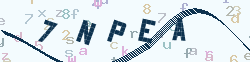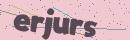# PHP开发API接口的图形验证码

2019-12-24 18:19:11API 接口，生成验证码并验证的方法其实很多，比如有利用 Redis / 缓存 开发。

BTW，该项目开发的时候，用的是 TP5 框架 使用 Postman 可以正常使用 Session 因为携带了 Cookie. 而博主在质疑接口使用 Session 的时候有用 Laravel 框架进行测试。发现接口并不能设置、获取到 Session. 所以如果该项目用的是 Laravel 框架的话，在前期就会放弃用 Session 而不会继续踩坑。

Laravel 的缓存系统还可以使用 Redis 作为驱动。如果不想要这样，可以直接读写 Redis 进行操作。

```public function getCaptcha()
{
\$imageW = 0;

\$length = 5;

\$fontSize = 25;

\$imageH = 0;

\$image = null;

\$bg = [243, 251, 254];

\$color = null;

\$fontttf = '';

\$useImgBg = false;

/********* 以上是配置项 *********/

// 图片宽(px)
\$imageW || \$imageW = \$length * \$fontSize * 1.5 + \$length * \$fontSize / 2;

// 图片高(px)
\$imageH || \$imageH = \$fontSize * 2.5;

// 建立一幅 \$imageW x \$imageH 的图像
\$image = imagecreate(\$imageW, \$imageH);

// 设置背景
imagecolorallocate(\$image, \$bg, \$bg, \$bg);

// 验证码字体随机颜色
\$color = imagecolorallocate(\$image, mt_rand(1, 150), mt_rand(1, 150), mt_rand(1, 150));

// 验证码使用随机字体
\$ttfPath = public_path('ttfs') . '/';

\$dir  = dir(\$ttfPath);
\$ttfs = [];

while (false !== (\$file = \$dir->read())) {
if ('.' != \$file && substr(\$file, -4) == '.ttf') {
\$ttfs[] = \$file;
}
}

\$dir->close();

\$fontttf = \$ttfs[array_rand(\$ttfs)];

\$fontttf = \$ttfPath . \$fontttf;

// 绘杂点
\$codeSet = '2345678abcdefhijkmnpqrstuvwxyz';
for (\$i = 0; \$i < 10; \$i++) {
//杂点颜色
\$noiseColor = imagecolorallocate(\$image, mt_rand(150, 225), mt_rand(150, 225), mt_rand(150, 225));
for (\$j = 0; \$j < 5; \$j++) {
// 绘杂点
imagestring(\$image, 5, mt_rand(-10, \$imageW), mt_rand(-10, \$imageH), \$codeSet[mt_rand(0, 29)], \$noiseColor);
}
}

// 绘干扰线
\$px = \$py = 0;

// 曲线前部分
\$A = mt_rand(1, \$imageH / 2); // 振幅
\$b = mt_rand(-\$imageH / 4, \$imageH / 4); // Y轴方向偏移量
\$f = mt_rand(-\$imageH / 4, \$imageH / 4); // X轴方向偏移量
\$T = mt_rand(\$imageH, \$imageW * 2); // 周期
\$w = (2 * M_PI) / \$T;

\$px1 = 0; // 曲线横坐标起始位置
\$px2 = mt_rand(\$imageW / 2, \$imageW * 0.8); // 曲线横坐标结束位置

for (\$px = \$px1; \$px <= \$px2; \$px = \$px + 1) {
if (0 != \$w) {
\$py = \$A * sin(\$w * \$px + \$f) + \$b + \$imageH / 2; // y = Asin(ωx+φ) + b
\$i  = (int)(\$fontSize / 5);
while (\$i > 0) {
imagesetpixel(\$image, \$px + \$i, \$py + \$i, \$color); // 这里(while)循环画像素点比imagettftext和imagestring用字体大小一次画出（不用这while循环）性能要好很多
\$i--;
}
}
}

// 曲线后部分
\$A   = mt_rand(1, \$imageH / 2); // 振幅
\$f   = mt_rand(-\$imageH / 4, \$imageH / 4); // X轴方向偏移量
\$T   = mt_rand(\$imageH, \$imageW * 2); // 周期
\$w   = (2 * M_PI) / \$T;
\$b   = \$py - \$A * sin(\$w * \$px + \$f) - \$imageH / 2;
\$px1 = \$px2;
\$px2 = \$imageW;

for (\$px = \$px1; \$px <= \$px2; \$px = \$px + 1) {
if (0 != \$w) {
\$py = \$A * sin(\$w * \$px + \$f) + \$b + \$imageH / 2; // y = Asin(ωx+φ) + b
\$i  = (int)(\$fontSize / 5);
while (\$i > 0) {
imagesetpixel(\$image, \$px + \$i, \$py + \$i, \$color);
\$i--;
}
}
}

// 绘验证码
\$code   = []; // 验证码
\$codeNX = 0; // 验证码第N个字符的左边距
for (\$i = 0; \$i < \$length; \$i++) {
\$code[\$i] = \$codeSet[mt_rand(0, strlen(\$codeSet) - 1)];
\$codeNX += mt_rand(\$fontSize * 1.2, \$fontSize * 1.6);
imagettftext(\$image, \$fontSize, mt_rand(-40, 40), \$codeNX, \$fontSize * 1.6, \$color, \$fontttf, \$code[\$i]);
}

// 验证码明文
\$plaintext_code = '';
foreach (\$code as \$this_code) {
\$plaintext_code .= \$this_code;
}

// 输出图像
ob_start();
imagepng(\$image);
\$content = ob_get_contents();
ob_end_clean();
imagedestroy(\$image);

return [
'content' => 'data:image/png;base64,' . base64_encode(\$content),
'code' => \$plaintext_code
];
}```

```{
"content": "data:image/png;base64,这里会是一串经过 Base64 编码的图形验证码",
"code": "hckkn"
}```

content 就是可以返回给前端的图形验证码编码。code 就是验证码的明文，可以将验证码明文存入 Redis.

`\$ttfPath = public_path('ttfs') . '/';`